International Journal of Mechanical Engineering and Applications
Volume 4, Issue 5, October 2016, Pages: 166-175

Dynamic Analysis and Design of Motorcycle Mounting System Subjected to Road Loads

Department of Mechanical Engineering, University of Wisconsin Milwaukee, Milwaukee, Wisconsin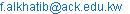(F. Alkhatib)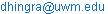(A. K. Dhingra)

*Corresponding author

Fadi Alkhatib, Anoop K. Dhingra. Dynamic Analysis and Design of Motorcycle Mounting System Subjected to Road Loads. International Journal of Mechanical Engineering and Applications. Vol. 4, No. 5, 2016, pp. 166-175. doi: 10.11648/j.ijmea.20160405.11

Received: July 30, 2016; Accepted: August 24, 2016; Published: September 10, 2016

Abstract: This paper presents a comprehensive model of a motorcycle mounting system. The model presented herein consists of two main assemblies. The powertrain assembly and the swing-arm assembly are modeled as a six degree of freedom rigid bodies. The two assemblies are connected to each other using a shaft that is usually referred to as the coupler. The connection points on both assemblies are known. Unlike automobiles, motorcycle performance and handling is highly affected by the external disturbance. In addition to minimizing the shaking loads, the mounting system must be set up such that it also minimizes the external disturbance from the environment such as irregularities in the road profile and road bumps. This disturbance can be transmitted through the tire patch to the engine causing it to hit nearby components. The engine movement needs to be minimized due to space limitations surrounding the engine. In order to do so, these transmitted external loads must be minimized by the use of the mounting system. The load minimization process is achieved by selecting the optimum stiffness parameters, location and orientation of the mounting system that are supporting the engine. This goal is achieved by an optimization scheme that guarantees that the transmitted loads are minimized. An investigation will be done to explore the effect of different road profiles on the mount final geometrical shape.

Contents

1. Introduction

In the paper presented herein, the effect of external loads on the mounting system is investigated. One of the main problems that engineers encounter in vibration isolation is the problem of motion isolation. This problem is seen in the case of external loads that are transmitted to the engine. These loads which are due to the irregularities of the road profile are transmitted to the frame through the tire patch. A periodic road profile will be investigated in this work. The main goal is to come up with an appropriate mounting system that minimizes the transmission of these external loads.

Since the powertrain represents the main lumped mass in a vehicle, it should be fixed on the frame using a resilient rubber mount. The mounting system is designed in a way that guarantees low vibration transmission from/into the engine. Internal loads and external loads or both are among the main source of vibration that should be considered. The shaking forces are generated due to the engine imbalance and the external load could be periodic or non-periodic. The work presented herein considers both periodic and non-periodic road profiles. Fig. 1, shows the twelve degree of freedom model used in the study where the powertrain is connected to the swing-arm via the coupler shaft. The engine mount plays a major role in isolating the vibrations transmitted due to various loads and supporting the powertrain static weight. It is also used to fix the powertrain in place and prevent it from any sort of movement in the fore-aft, lateral and vertical directions. In order to come up with a proper mounting system, finding the mount characteristics only are not sufficient.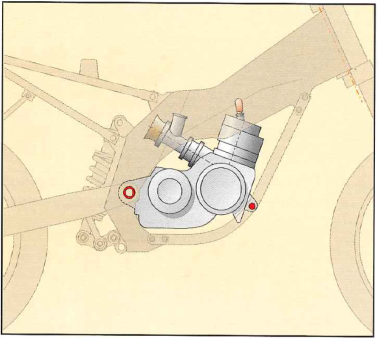Figure 1. Twelve DOF model.

The issue of minimizing forces that are transmitted through the mounting system is discussed by . These forces can be caused as a result of rotational imbalance and reciprocating masses. The design of engine mount has been addressed by . He suggested treating the mount orientation and stiffness as variables. Another suggestion presented by  that incorporates the mount geometric properties as well. In the work presented herein, a shear (bush) mount which is made of rubber is used. This type of mounts is commonly used in passenger cars due to its low cost. These mounts may vary in shape depending on the specifications of the design. In the design process the geometry is parameterized to define the shape of the mount. The parameters are found by optimizing the minimum difference of the stiffness values and the desired stiffness values obtained from vibration analysis. The optimization process is done using a nonlinear finite element model that employs the nonlinear properties of rubber from which the mount is made off. A method used in the optimization design of engine mounts is presented in . The constraint problem is solved using some of the known parameters such as engine center of gravity, mount stiffness rates and mount location and/or orientation. A new technique to find an optimized and robust solution for the mounting system is suggested in . Multi objective algorithm (Pareto optimization) is used as a base to the multi objective robust optimization problem. The use of this technique enhances the vehicle isolation characteristics. A study of the dynamic analysis and parameter identification of a rubber isolator using Maxwell-Voigt model is presented in . In the study, they noticed the difference between the Voigt model which simply consists of a spring damper connected in parallel and the Maxwell-Voigt model which includes another spring and a damper connected in series the Maxwell model.

This paper introduces a comprehensive twelve degree of freedom model for the motorcycle mounting system taking into consideration the effect of the road loads. Road loads are critical when designing a motorcycle mounting system and this due to the nature of motorcycles and what it takes to achieve the best handling. This paper also introduces the concept of mount shape optimization in which the final mount shape depends on the stiffness values of the mounts in all three principal directions.

The paper is organized as follows. Section 2 discusses the dynamic analysis in which the engine mount is characterized. Section 3 explains the road loads and the road profiles. In this section, the external load transmitted to the engine through the tire patch is formulated. The optimization problem formulation is presented in section 4. The concept of shape optimization is presented in section 5. Section 6 and section 7 presents the numerical results and conclusions.

2. Dynamic Analysis

This section represents the equations of motion of the mounting system. Fig. 2, shows a schematic diagram of the powertrain, swing-arm, rear shock absorber and the coupler. The complete model used herein consists of two rigid bodies. The first rigid body represents a six degree of freedom model of the powertrain and the second rigid body represents another six degree of freedom model of the swing-arm. The connection between the two rigid bodies is done using a coupler shaft that is modeled as a spring-damper system with translational and rotational capabilities in the three coordinate directions. The rear shocks and the rear wheel are modeled as a spring-damper element. Fig. 3, shows a schematic diagram of the mount that will be used in the study presented herein. The model represented in Fig. 3 is a simple Voigt model that consists of a rigid body that resembles the powertrain which is connecting to the frame using the mounting system. The stiffness k and the damper c represent a single D.O.F system with an equation of motion shown in Eq. (1).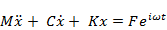(1)

In Eq. (1), M, C and K represent the system mass, damping and stiffness matrices respectively. F denotes the input force vector that can be caused be either the shaking force or the road load or both. x represents the displacement vector. The terms of the inertia matrix M of the powertrain are with respect of the global coordinate system. The engine mounts stiffness, location and orientation are presented in the mount local coordinate system. The mount parameters must be transformed using a transformation matrix to the global coordinate system. The mass matrix of the powertrain is represented in Eq. (2).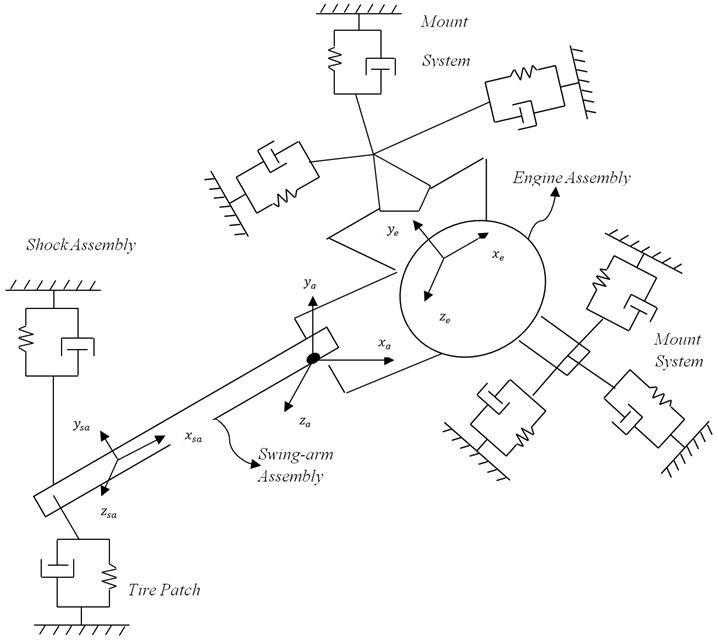Figure 2. Powertrain and swing-arm layout.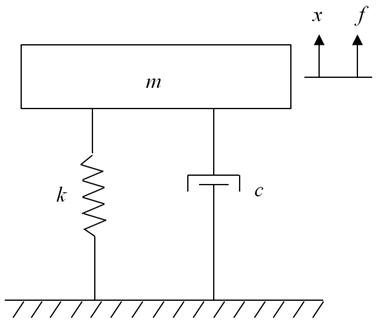Figure 3. Schematic diagram of the mount model.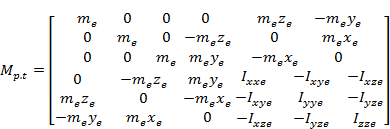(2)

In Eq. (2), Mp.t is the mass of the powertrain assembly, (xe, ye, ze) is the location of the center of gravity of the powertrain with respect to the origin of the coordinate system and Ixxe, Iyye, Izze, … are the inertia of the powertrain with respect to the origin of the coordinate system. The stiffness and damping matrices of an individual mount expressed about its own coordinate system is given by Eqs. (3) and (4).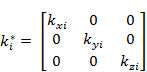(3)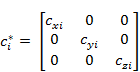(4)

A transformation matrix (A) is used in order to transfer both, the stiffness and damping matrices to the global coordinate system as follows: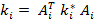and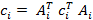where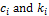are the individual mount damping and stiffness matrices expressed in the global coordinate system. The matrix Ai is a transformation matrix which is a combination of the three different rotations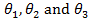about x, y and z axes with respect to the global coordinate system.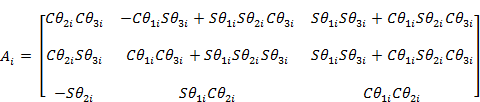(5)

In Eq. (5)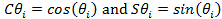.

The transformed damping and stiffness matrices are shown in Eq. (6)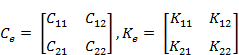(6)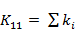,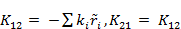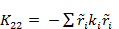(7)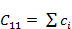,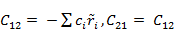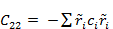(8)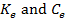represents the overall damping and stiffness matrices of the powertrain assembly shown in Eq. (6).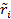represents the skew-symmetric matrix that corresponds to an individual mount position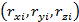and it’s given by: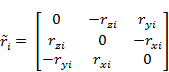(9)

A comprehensive twelve DOF model will be used to represent the engine mounting system. A coupler shaft is used to connect the powertrain assembly and the swing-arm assembly at a pivot point. This configuration is common in motorcycle applications and provides sufficient information to capture the isolation characteristics. This model is based on two rigid bodies, one is for the powertrain assembly and the other one is for the swing-arm assembly connected together using a coupler shaft. This model assumes that the frame is infinitely rigid. The equation of motion of the twelve DOF system is as follows: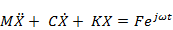(10)

In Eq. (10), M, C and K are a 12 x 12 mass, damping and stiffness matrices respectively of the coupled swing-arm powertrain assembly. X is the displacement vector of the assembly which consists of the translational and rotational displacements.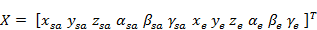The mass, stiffness and damping matrices representing the two rigid bodies, i.e. the powertrain and the swing-arm are constructed separately and then combined using the coupler shaft properties. The subscript ‘sa’ represents parameters related to the swing-arm and the subscript ‘e’ represents parameters related to the powertrain. To account for different orientations of the mounts, the stiffness and damping are compiled in the mount local coordinate system then transformed using the transformation matrix shown in Eq. (5). Eq. (5) consists of a set of rotational matrices about the three coordinates. Bryant angles, Euler angles or directional cosines could be used to construct the transformation matrix.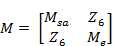(11)

In Eq. (11), Z6 is 6x6 zero matrix and Msa and Me are 6x6 swing-arm and powertrain inertia matrices. The stiffness and damping matrices of the assembly are defined as follows: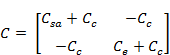(12)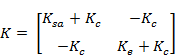(13)

In Eqs. (12) and (13), Ksa, Ke, Csa and Ce are 6x6 stiffness and damping matrices of the swing-arm and powertrain. Ksa and Csa are constructed that same way that Ke and Ce are constructed as shown in the previous section. Kc and Cc are 6x6 diagonal matrices that represent the stiffness and damping of the coupler that connects the swing-arm and powertrain.

The road loads are due to irregularities in the road profile which could be periodic or non-periodic. These road profiles are analyzed for a specific displacement functions in which the frequency content of the periodic profiles is determined using the Fourier series expansion of the displacement function. The frequency content for the non-periodic profiles is determined using the Fourier transform. Herein, the Fourier series coefficient and the frequency content are obtained using the Fast Fourier Transforms (FFT) presented in . The input force resulted from a certain road profile is determined using Eq. (14).(14)

In Eq. (14), Fy is the vertical component of the force that is transmitted through the tire patch due to the displacement x and the velocity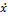as a result of the road profile change. k and c are the stiffness and damping of the rear wheel in the y-direction.

The continuous time Fourier series (CTFS) for a periodic road profile is represented as follows: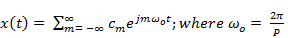(15)

cm represents the Fourier series coefficients and are determined using Eq. (16).(16)

In the above equations P is the fundamental period of the displacement function x(t) that corresponds to the fundamental frequency ωo. On the other hand, the discrete time Fourier series (DTFS) is represented for the discredited displacement function as follows: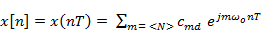(17)

In Eq. (17)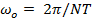and cmd are the Fourier series coefficients which are determined as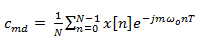(18)

In Eqs. (17) and (18), ωo is the fundamental frequency and T is the sampling period. The DTFS coefficients can be determined using Eq. (19), if the a band limited displacement function x(t) and an appropriate sampling period T is chosen using FFT.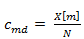(19)

In Eq. (19), X[m] is the FFT of x[n] and N is the number of terms of x[n] used to compute the FFT.

The continuous time Fourier transform (CTFT) of the displacement function is given in Eq. (20) and the discrete time Fourier transform (DTFT) is given in Eq. (21).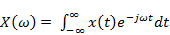(20)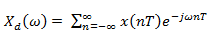(21)

In the above equations, X(ω) is the spectrum of x(t) which can used for periodic and non-periodic displacement functions.

Fast Fourier transform (FFT) is employed herein to analyze the road input for which the displacement profile is known as a function of time as shown in Fig. 4. For these inputs, the road load is modeled as shown in Eq. (14). The model presented in Eq. (14) is a simplistic model that holds in the cases where no slip is present. FFT is used to obtain the frequency spectrum for the road profile. Truncation of the spectrum and the time history are shown in Figs. 5 and 6 respectively which are used to reconstruct the road profile shows the accuracy of the truncated model. This truncated spectrum is used to solve for the steady state displacement.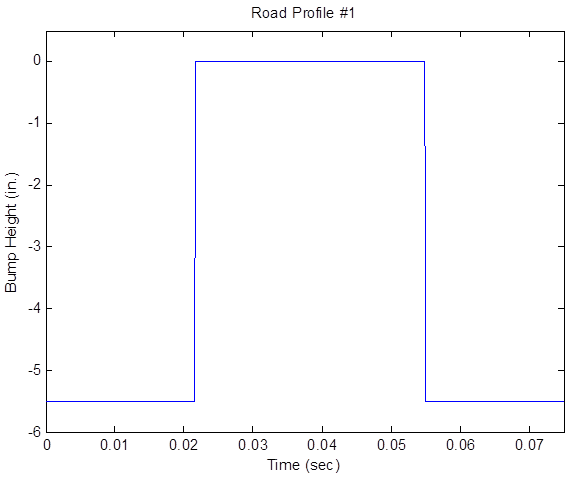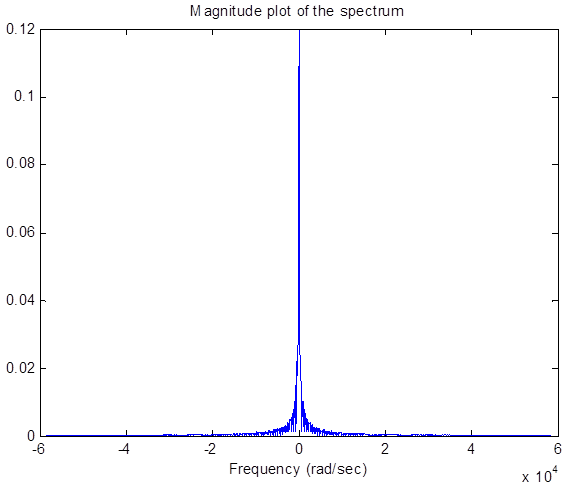Figure 5. Magnitude Spectrum.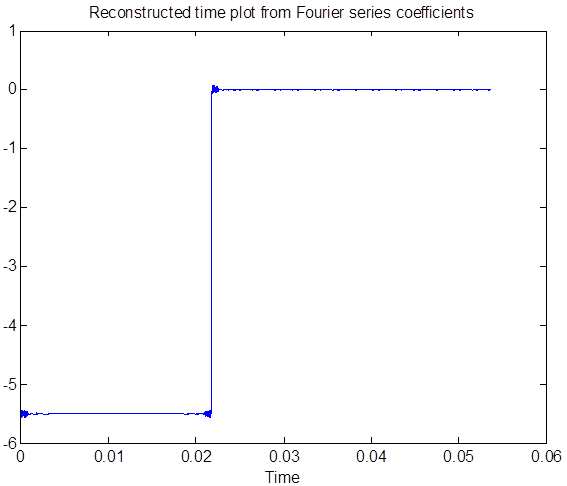Figure 6. Reconstructed Time Plot.

4. Optimization Problem

This section presents the formulation of the optimization problem. The mount optimization is highly non-linear. Multiple runs are done to avoid premature convergence to local minima and multiple starting points were used. The optimization problem for is perused using the Sequential Quadratic Programming (SQP) technique presented in . The objective function that is used in this work is the weighted sum of the transmitted force through the individual mount. The transmitted forces through the mounts are due to the irregularities in the rood profile and road bumps. The force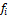transmitted to the frame through the individual mount is given as follows: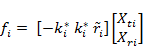(22)

In Eq. (22),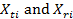represents the translational and rotational displacement at the center of gravity of the powertrain as result of the shaking load.is the local stiffness matrix for the individual mount andis the skew symmetric from the position vector of the individual mount represented in Eq. (9). The objective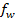function is assembled by summing the Euclidean norm of the individual force transmitted through each mount.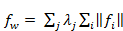(23)

In Eq. (23),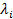is the weighting parameter that corresponds to different loading conditions.

Deflection constraint is added to the optimization problem which dictates the maximum allowable engine weight. The static deflection Xst at the origin of the global coordinate system is computed as: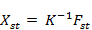(24)

In Eq. (24), Fst is the static load acting on the system.

The engine mount optimization problem can be stated as follows: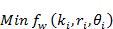(25)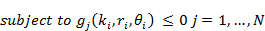In Eq. (25), the mount stiffness, location and orientation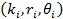are the design variables that are subjected to a total of N number of constraints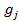. The constraints that are used in the above problem consist of bounds constraints on the engine mount stiffness, constraints on the mount location based on the available space, constraints on the mount orientation that is dictated by symmetry and finally a constraint on the deflection of the center of gravity of the powertrain due to the static weight of the powertrain. The objective function fw is defined in Eq. (23). Both fw andare functions of the design variables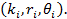5. Shape Optimization

The geometric dimensions of the isomeric mount shown in Fig. 7 are determined via a parametric study. These optimum values for the dimensions are chosen such that a complete discerption of the mount is achieved. The mount final shape is determined by minimizing the difference between the mount stiffness values obtained from the dynamic analysis performed in the previous section and the mount stiffness values based on its geometry which can be found from the finite element analysis. The objective function that is employed herein is described in Eq. (26) must satisfy alongside with the bound on the design variables the condition described in Eq. (27), where xi is the ith design variable and n is the number of the design variables stated in .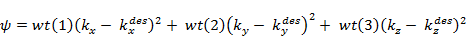(26)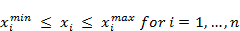(27)

In Eq. (26),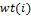is the weighting function that corresponds to the stiffness in the ith direction. The superscript ‘des’ indicates the desired stiffness that is obtained from the dynamic analysis of the mounting system, meanwhile the design parameters selected will determine the stiffness values for the geometry that is obtained from the nonlinear finite element analysis. The process of determining the design variables is expensive and time consuming, therefore in order to reduce the number of function evaluations, the least effective stiffness could be dropped from the objective function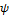.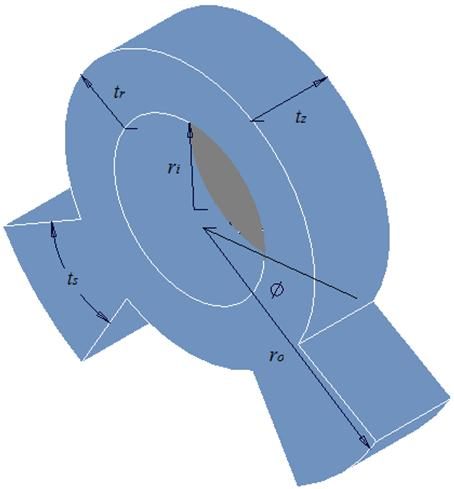Figure 7. Schematic of a rubber mount.

Figure 7, shows the actual geometry of an engine mount that is used in cars along with its defining parameters. This mount is a bush type that is made of rubber. There are a total of six parameters that dictates the shape of the mount in which four are used as the design variables namely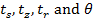. The other two parameters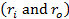are constants. These design variables affects the mount stiffness directly. The weighting function that is used in the objective function could be used to take into account the importance of the stiffness in a particular direction. The dynamic analysis is done for a motorcycle powertrain in which is supported by four isomeric mounts. The connection between the powertrain and the swing-arm are taken into consideration generating a twelve DOF system. The exciting force is due to the internal shaking force at 4000 rpm and the road load presented in Fig. 4.

In this work, the stiffness values are obtained using a nonlinear finite element analysis. The geometry shown in Fig. 7 is used to generate a mesh for the analysis. The optimization is carried out using ANSYS. Solid 186 is the element that has been used for this purpose. Appropriate boundary conditions has been applied to the model which is assumed to exhibit small deflections, for this reason the Mooney Rivlin model is sufficient to describe the fully incompressible hyperelastic material behavior of rubber presented by  and . The Mooney Rivlin model of the strain energy is expressed as: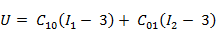(28)

I1 and I2 are the first and second strain invariants. The coefficients C10 and C01 are determined from the uniaxial tension test. The rubber that is used in this work is carbon black filled natural rubber. The values of the coefficients are:

C10 = 0.03622 and C01 = -0.00335.

All the design variables must satisfy the design range which could be considered as inequality constraints that dictates the lower and upper bound of these variables. Each one of these ranges that specify the upper and lower limit of the design variables are considered as inequality constraints and are incorporated in the finite element optimizer. The static deflection that is due to the static weight of the engine is measured along the axis of gravity.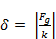(29)

Fg represents the static weight of the engine due to gravity and k represents the stiffness in the gravity direction.

6. Numerical Example

The mounting system presented in this example consists of four identical circular cross section elastomeric mounts with symmetry constraints. Two of the engine mounts are at the front of the powertrain assembly and the other two are located at the rear of the powertrain assembly as shown in Fig. 8. A loss factor of 0.3 and dynamic to static stiffness ratio of 1.2 has been used for all the mounts. The powertrain and the swing-arm data is given in Table 1. The swing-arm is connected to the frame using two shock absorbers which are inclined by an angle of 47° with respect to the horizontal axis. The shock absorber exhibits an axial stiffness and damping of 45 lb/in and 4.4 lb-s/in respectively. The stiffness of the coupler used in the example is 42655 lb/in in the x and y direction and 658252 lb/in along the z axis. The rotational stiffness values is 682493 lb-in/rad about the x and y axes. The rotational stiffness about the z axis is zero.

In this example, the force transmitted through the engine mounts due to both the shaking force at 4000 rpm and the road loads described in the profile shown in Fig. 4, are used to formulate the objective function shown in Eq. (23). The design vector contains the individual mount stiffness, orientation and position. Lower and upper bound for the design variables are listed in Table 2. Deflection constraints on the powertrain are considered due to the static and dynamic loads. The static constraints which are placed on the deflection of the powertrain are as follows: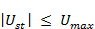(30)

In Eq. (30), Ust represents the static deflection of the powertrain at its C.G. due to the static load and Umax represents the maximum allowable deflection due to the static load. For this example. An additional constraint is placed on the maximum displacement at the mount location in the y direction of 0.05 in to prevent premature snubbing. The shaking force at 4000 rpm and the static load vector due to the engine weight are given by Eq. (31) and Eq. (32) respectively.Figure 8. Mount System Layout.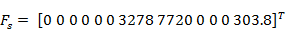(31)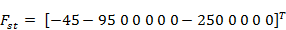(32)

The optimization problem is done using the SQP technique that employs a function to minimize the value of the objective function. Once the operation is over, the design vector that corresponds to the optimum value of the objective function is known. These results are shown in Table 3. The second part of the problem starts by setting the objective function described in Eq. (26) to minimize the difference between the desired stiffness values obtained from the first optimization done through the dynamic analysis and the stiffness values obtained from the geometric shape of the mount. Since the stiffness of the steel plates is higher than the mount stiffness, the constraints are moved from the plate holes directly into the mount surface as shown in Fig. 9. The boundary conditions are applied by constraining the displacement of the surface of the mount in all directions. The results of the shape optimization process are shown in Table 4. The shape optimization takes into account the range of the design variables that acts like lower and upper bounds. These bounds are shown in Eq. (33) and the starting shape of the mount and the optimized shapes are shown in Figs. 10 and 11.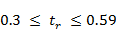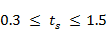(33)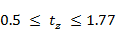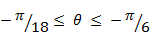The mount stiffness that is used in the design vector in the optimization problem is the dynamic stiffness. The elastomeric parameters of the engine mount can be determined using the dynamic stiffness which is obtained from the optimization problem. The dynamic stiffness of the elastomeric mount is defined in Eq. (34).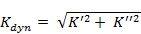(34)

In Eq. (34), Kdyn is the dynamic stiffness of the mount,are the real and complex components of the dynamic stiffness. The ratio of the dynamic stiffness, Kdyn, to the static stiffness Kst, is known as the dynamic-to-static stiffness ratio and is always greater than one. Another parameter that is used to characterize the engine mount is the loss factor. The loss factor is defined in Eq. (35) and is used to provide a measure of damping.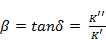(35)

The damping coefficient, c, of an elastomeric mount is defined in Eq. (36)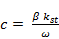(36)

In Eq. (36),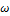is the input excitation frequency. The higher the dynamic-to-static stiffness ratio, then dynamic stiffness will be higher. However, since the frequency range of interest for this study is small, the frequency dependence of stiffness has been ignored. This is because the mount optimization is performed at a specific cruising speed (4000 rpm), and the proving ground test-track road load yields high amplitude, low frequency loads.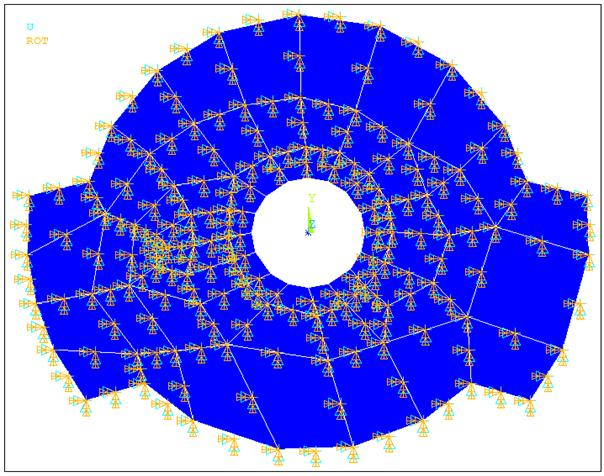Figure 9. Front View Showing the Boundary Conditions.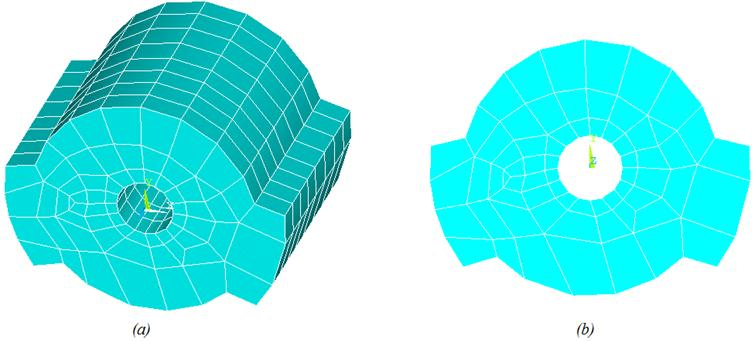Figure 10. (a) Isometric View of the Initial Geometry, (b) Front View of the Initial Geometry.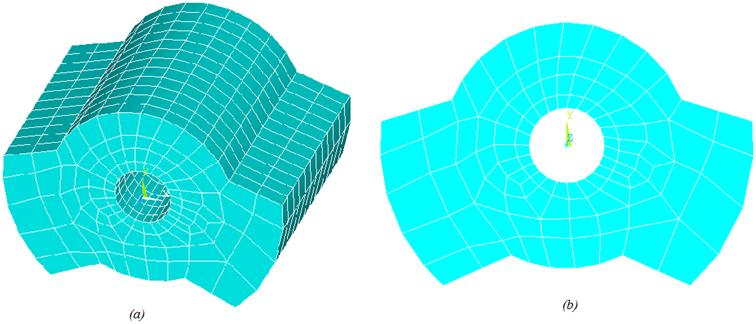Figure 11. (a) Isometric View of the Optimized Geometry, (b) Front View of the Optimized Geometry.

Table 1. Swing-arm and Powertrain Data with Respect to Local C.G.

 Powertrain Swing-arm Mass lb-s2/in 0.5 0.13 Ixx lb-in2 20.7 0.465 Iyy 12.81 30 Izz 26.14 29 Ixy 1.86 0.002 I2xz 0.12 -0.007 Iyz 2.3 -0.008

Table 2. Bounds for Design Variables.

 Min. Max. Mount Stiffness (x, y) lb/in 100 5000 Mount Stiffness (z) 500 15000 Orientation Angles deg. 0 50

Table 3. MatLab Optimization Results.

 Load Transmitted Mount Stiffness (lb/in) (lb) x y z Initial Guess 540.77 4750 4750 2400 Optimized Design 244.74 1618 1618 15000

Table 4. Parameter Optimization Results.

 Initial Optimized Target Stiffness Design Variables θ (rad) 6.021 5.923 tr (in) 0.591 0.454 ts (in) 0.787 0.965 tz (in) 1.378 1.430 Stiffness (klb/in) kx 3.411 1.6192 1.618 ky 9.183 1.6185 1.618 kz 1.852 15.085 15 Obj. Function ψ 233.31 0.0072

7. Conclusion

The final shape of a shear (bush) type engine mount has been achieved through the process of parameterization of the engine mount geometrical properties utilizing a nonlinear finite element analysis. Phase one of the design was done using the SQP method provided by MatLab built in function in order to find the target stiffness values. In this phase multiple initial guess have been used in order to ensure that the optimum value of the objective function is a global minima and not local. This problem is due to the fact that the engine mount optimization problem is nonlinear and local minimum values of the objective function are quite possible. As it can be seen from the results shown in figs. 6 and 7, the optimum mount shape is acceptable and can be considered as the final shape. The stiffness values that are obtained from the shape optimization process are very close to the target stiffness values obtained from the dynamic analysis. The final shape is acceptable and can be used in real mount design situations. It is worth mentioning that this approach is applicable to any type of engine mounts.

References

1. Aikawa Y, Osakabe T, Sunayama Y. (2005) Prediction of engine mount vibration using multi body simulation with finite element models. SAE Small Engine Technology Conference, Paper # 2005-32-006.
2. ANSYS help documentation (2009), version 12, ANSYS Inc.
3. Chen CT (2001) Digital Design Processing – Spectral Computation and Filter Design, Oxford University Press.
4. Courteille E, Mortier F (2005) Multi-Objective Robust Design Optimization of an Engine Mounting System. SAE, paper no. 01-2412.
5. Kaul, S., Modeling Techniques for Vibration Isolation in Motorcycles, PhD. Thesis, University of Wisconsin, Milwaukee, 2006.
6. Kim J, Kim Y (1997) Shape Design of an Engine Mount by a Method of Parameter Optimization. Computers and Structures, Vol. 65, No. 5: 725-731.
7. Liu C Q (2003) A Computerized Optimization Method of Engine Mounting System. SAE, paper no. 01-1461.
8. MATLAB User Guide (2010), Version 7.10, Math Works.
9. Norton RL (2011) Design of Machinery: An Introduction to the Synthesis and Analysis of Mechanisms and Machines, 5th edition. McGraw Hill.
10. Pacejka HB (2002) Tyre and Vehicle Dynamics, Butterworth-Heineman.
11. Paul B (1979) Kinematics and Dynamics of Planner Machinery, Englewood Cliffs, NJ: Prentice Hall Inc.
12. Rao SS (2009) Engineering Optimization Theory and Practice, 4th edition. New York, NY: John Wiley & Sons.
13. Rivlin RS (1992) The Elasticity of Rubber. Rubber Chem Technol 65: G51-G66.
14. Snyman JA, Heyns PS, Vermenulen PJ (1995) Vibration Isolation of a Mounted Engine Through Optimization. Mechanical and Machine Theory, Vol. 30, No. 1, pp. 109-118.
15. Spiekermann CE, Radcliff CJ, Goodman ED (1985) Optimal Design and Simulation of Vibrational Isolation Systems. Journal of Mechanisms, Transmission and Automation in Design, Vol. 107, pp. 271-276.
16. Swanson SR (1985) Large Deformation Finite Element Calculations for Slightly Compressible Hyperelastic Materials. Computers and Structures, Vol. 21, pp. 81-88.
17. Zhang J, Richard MC (2006) Dynamic Analysis and Parameter Identification of a Single Mass Elastomeric Isolation System Using a Maxwell-Voigt Model. Journal of Vibration and Acoustics, Vol. 128, pp. 713-721.

 Contents 1. 2. 3. 4. 5. 6. 7.
Article ToolsAbstractPDF(652K)